Courses
Courses for Kids
Free study material
Free LIVE classes
More

# Rectilinear ShapesLIVE
Join Vedantu’s FREE Mastercalss

## What is a Rectilinear Shape?

Any shape that you see around yourself has straight sides or edges is rectilinear. Let us assume that you are learning to drive a car for the first time. Now, as you are new to all the systems like brake, accelerator, and clutch, you need to learn to take your car ahead.

As soon as you get some hold on the ABC of the car, you need a straight road that is free of diversion so that you are comfortable to move it ahead. Now, what path have you taken while learning to drive? Well, it is a rectilinear path or a straight path.

A question arises why do we learn this concept in maths and what other properties does any shape carry while making sure that the shape is rectilinear. So, let us focus on this part!

## Rectilinear Shapes in Maths

From the above text, we have got an idea of what rectilinear is, now, let us understand it through the following shapes:

Let us suppose that your friend gifted you a video game packed in a rectangular big box. Now, this rectangular box has straight edges or sides.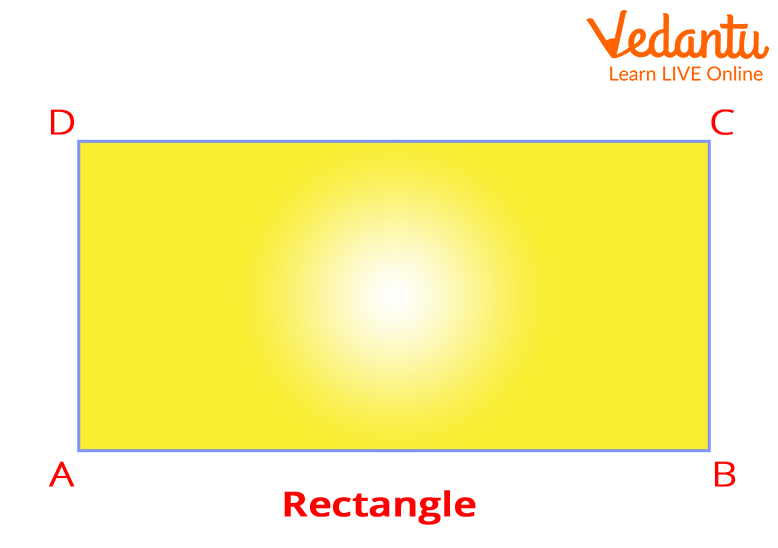Also, between each side, there is a corner. Marking these four corners as A, B, C, and D. However, we notice that an angle between sides BA and DA or angle BAD is a right angle. Similarly, we notice in the pairs AB and CB, i.e., angle ABC = 90 degrees, BC and DC or angle BCD = 90 degrees, and lastly, we have DA and CD, i.e., angle ADC = 90 degrees.

We conclude one more thing from our above explanation that a rectilinear shape has straight edges with angular corners (here angular corners are 90 degrees).

Important Point:

Please note that rectilinear means straight like you can have a straight path or motion. Also, interior angles in these shapes are 90-degrees, or it can be 270-degrees as well.

So, the concept of rectilinear shapes is not limited to quadrilaterals (polygons of shapes having “quad” or “four” sides and angles); it also well suits other polygons as well. Let us know how.

## Rectilinear Figures

Look at the below polygons that have rectilinear shapes:

1. Pentagon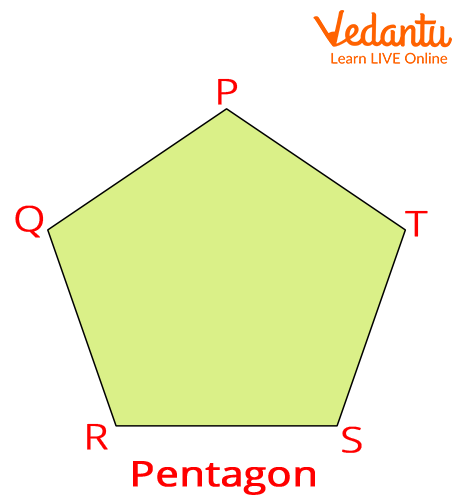1. Hexagon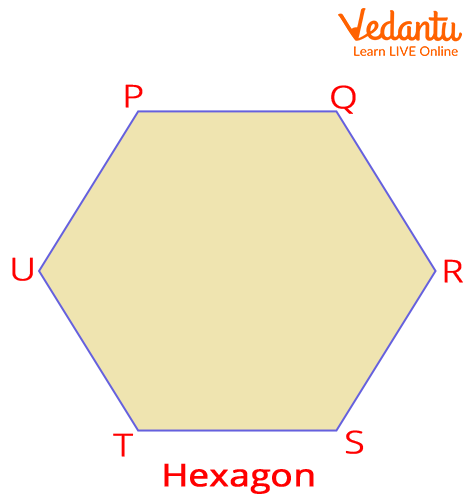1. Heptagon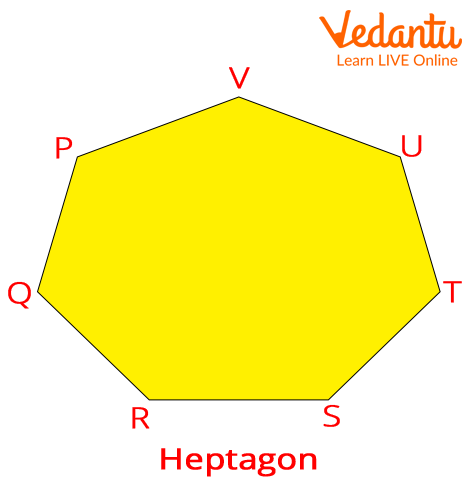1. Octagon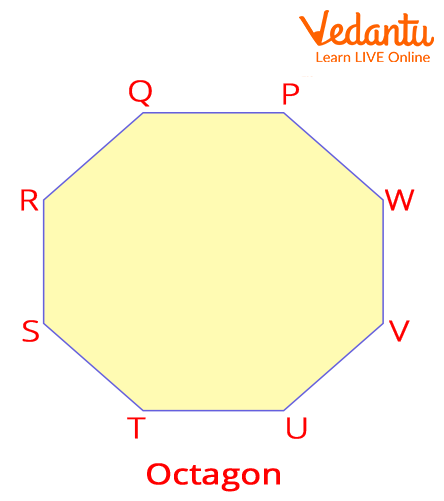1. Nonagon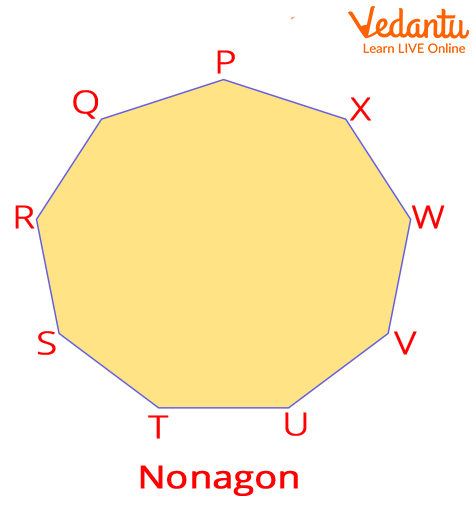1. Decagon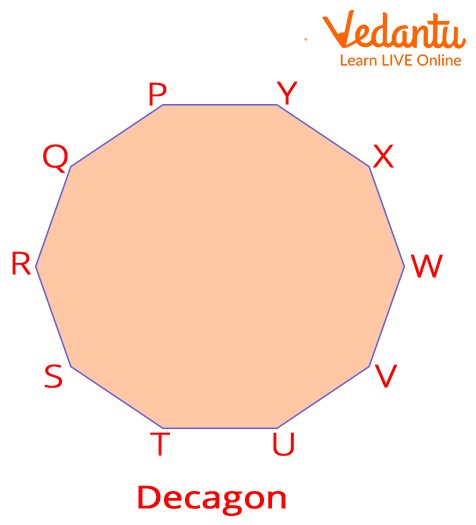So, this was all about rectilinear concepts, shapes, and figures having straight sides and angular corners. Now, let us go through some FAQs on this topic.

Last updated date: 29th Sep 2023
Total views: 141.3k
Views today: 3.41k

## FAQs on Rectilinear Shapes

1. Is a circle a rectilinear shape?

No, a rectilinear shape has straight lines and angular corners, where a circle doesn't hold either of the properties, which makes it pretty clear to us that a circle is not a rectilinear figure.

2. Are all polygons rectilinear figures?

Yes, every polygon is a rectilinear figure but every rectilinear figure is not a polygon. Besides this, an angle formed by two consecutive sides of a polygon is called an interior angle, an angle of the polygon.